Courses

# Test: Deflection of Beams - 2

## 10 Questions MCQ Test Topicwise Question Bank for Civil Engineering | Test: Deflection of Beams - 2

Description
This mock test of Test: Deflection of Beams - 2 for Civil Engineering (CE) helps you for every Civil Engineering (CE) entrance exam. This contains 10 Multiple Choice Questions for Civil Engineering (CE) Test: Deflection of Beams - 2 (mcq) to study with solutions a complete question bank. The solved questions answers in this Test: Deflection of Beams - 2 quiz give you a good mix of easy questions and tough questions. Civil Engineering (CE) students definitely take this Test: Deflection of Beams - 2 exercise for a better result in the exam. You can find other Test: Deflection of Beams - 2 extra questions, long questions & short questions for Civil Engineering (CE) on EduRev as well by searching above.
QUESTION: 1

### For cantilever beam shown in the figure, the deflection at C due to a couple M applied at Bis equal to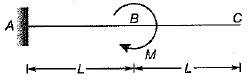Solution:

Slope and deflection at B,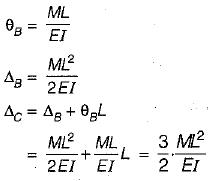[As there is no moment between B and C so BC remains linear at θB slope]

QUESTION: 2

Solution:
QUESTION: 3

### Which of the foilowing statements are correct. Macaulay's method for calcuiation of slope and deflection in a beam is suitable for 1. Prismatic beams only. 2. Several concentrated loads and can be extended to uniformly distributed loads. 3. Both prismatic and Non-prismatic beams,

Solution:

Macaulay’s method is based on singularity function. It is applicable for prismatic beams only. While Mohr’s moment area method can be used for prismatic and non-prismatic beams.

QUESTION: 4

A simply supported beam of span L and depth dcarries a central load W. The ratio of maximum deflection to maximum bending stress is

Solution: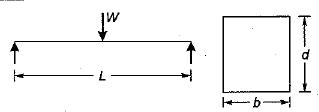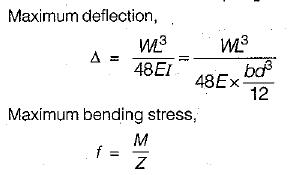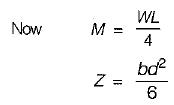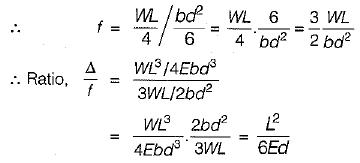QUESTION: 5

Calculate the reaction at the roller support for the cantilever beam shown in the figure?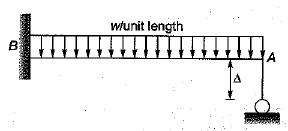Solution:

Let P be the roller reaction.
Upward deflection of beam at A due to P.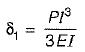Downward deflection of beam at A due to w.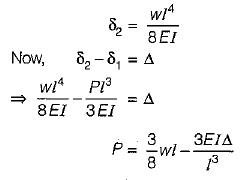QUESTION: 6

A cantilever carries a load P as shown in the given figure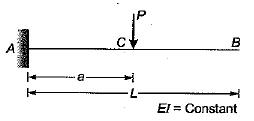The deflection at B is

Solution: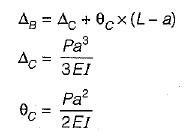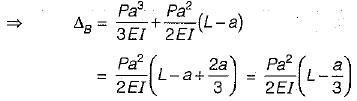QUESTION: 7

A simply supported beam of uniform flexural rigidity is loaded as shown in the given figure. The rotation at the end A is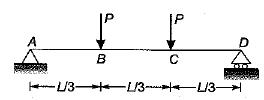Solution:

The M/EI diagram of beam is,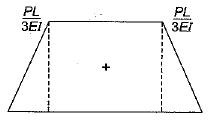The slope at mid span is zero.

The difference between the slope at two points is  M/EI area between these points.

So slope at A,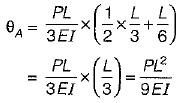QUESTION: 8

The maximum deflection of simply supported beam occurs at zero

Solution:
QUESTION: 9

The stepped cantilever- is subjected to moments, M as shown in the figure below. The vertical deflection at the free end ( neglecting self weight)  is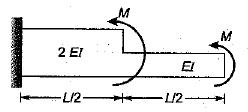Solution:

Using moment area method,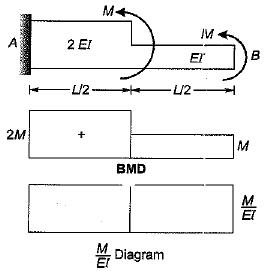Deflection at B, wrt A
= Moment of area of M/EI diagram between A and B about B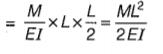QUESTION: 10

A simply supported beam of length CL' carries two equal unlike couples. M at the two ends. The central deflection of the beam is given by

Solution: